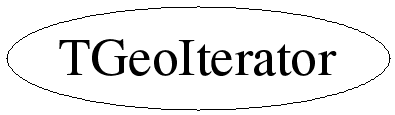# class TGeoIterator

``` TGeoIterator

A geometry iterator that sequentially follows all nodes of the geometrical
hierarchy of a volume. The iterator has to be initiated with a top volume
pointer:

TGeoIterator next(myVolume);

One can use the iterator as any other in ROOT:

TGeoNode *node;
while ((node=next())) {

}

The iterator can perform 2 types of iterations that can be selected via:

next.SetType(Int_t type);

Here TYPE can be:
0 (default) - 'first daughter next' behavior
1           - iteration at the current level only

Supposing the tree structure looks like:

TOP ___ A_1 ___ A1_1 ___ A11_1
|       |        |___ A12_1
|      |_____A2_1 ___ A21_1
|                |___ A21_2
|___ B_1 ...

The order of iteration for TYPE=0 is: A_1, A1_1, A11_1, A12_1, A2_1, A21_1,
A21_2, B_1, ...
The order of iteration for TYPE=1 is: A_1, B_1, ...
At any moment during iteration, TYPE can be changed. If the last iterated node
is for instance A1_1 and the iteration type was 0, one can do:

next.SetType(1);
The next iterated nodes will be the rest of A daughters: A2,A3,... The iterator
will return 0 after finishing all daughters of A.

During iteration, the following can be retreived:
- Top volume where iteration started:    TGeoIterator::GetTopVolume()
- Node at level I in the current branch: TGeoIterator::GetNode(Int_t i)
- Iteration type:                        TGeoIterator::GetType()
- Global matrix of the current node with respect to the top volume:
TGeoIterator::GetCurrentMatrix()

The iterator can be reset by changing (or not) the top volume:

TGeoIterator::Reset(TGeoVolume *top);

Example:

We want to find out a volume named "MyVol" in the hierarchy of TOP volume.

TIter next(TOP);
TGeoNode *node;
TString name("MyVol");
while ((node=next()))
if (name == node->GetVolume()->GetName()) return node->GetVolume();

```

## Function Members (Methods)

public:
 TGeoIterator(TGeoVolume* top) TGeoIterator(const TGeoIterator& iter) virtual ~TGeoIterator() static TClass* Class() const TGeoMatrix* GetCurrentMatrix() const Int_t GetIndex(Int_t i) const Int_t GetLevel() const TGeoNode* GetNode(Int_t level) const void GetPath(TString& path) const TGeoVolume* GetTopVolume() const Int_t GetType() const virtual TClass* IsA() const TGeoNode* Next() TGeoNode* operator()() TGeoIterator& operator=(const TGeoIterator& iter) void Reset(TGeoVolume* top = 0) void SetTopName(const char* name) void SetType(Int_t type) virtual void ShowMembers(TMemberInspector& insp, char* parent) void Skip() virtual void Streamer(TBuffer& b) void StreamerNVirtual(TBuffer& b)
protected:
private:
 void IncreaseArray()

## Data Members

private:
 Int_t* fArray Array of node indices for the current path Int_t fLevel Current level in the tree TGeoHMatrix* fMatrix Current global matrix Bool_t fMustResume Private flag to resume from current node. Bool_t fMustStop Private flag to signal that the iterator has finished. TGeoVolume* fTop Top volume of the iterated branch TString fTopName User name for top Int_t fType Type of iteration

## Class Charts## Function documentation

TGeoIterator(TGeoVolume *top)
``` Geometry iterator for a branch starting with a TOP node.
```
TGeoIterator(const TGeoIterator &iter)
``` Copy ctor.
```

``` Destructor.
```
TGeoIterator & operator=(const TGeoIterator& iter)
``` Assignment.
```
TGeoNode * Next()
``` Returns next node.
```

``` Returns next node.
```
const TGeoMatrix * GetCurrentMatrix()
``` Returns global matrix for current node.
```
TGeoNode * GetNode(Int_t level) const
``` Returns current node at a given level.
```
void GetPath(TString& path) const
``` Returns the path for the current node.
```
void IncreaseArray()
``` Increase by 30 the size of the array.
```
void Reset(TGeoVolume* top = 0)
``` Resets the iterator for volume TOP.
```
void SetTopName(const char* name)
``` Set the top name for path
```
void Skip()
``` Stop iterating the current branch. The iteration of the next node will
behave as if the branch starting from the current node (included) is not existing.
```
Int_t GetIndex(Int_t i) const
`{return 0;}`

`{ }`

`{return fLevel;}`

`{return fTop;}`

`{return fType;}`
void SetType(Int_t type)
`{fType = type;}`

Author: Andrei Gheata 24/10/01
Last update: root/geom:\$Id: TGeoNode.h 21494 2007-12-19 15:50:40Z brun \$